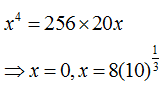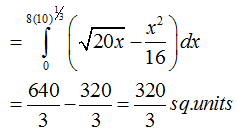# Topic: Application of Integrals (Test 1)

Topic: Application of Integrals
Q.1
For which of the following values of m , is the area of the region bounded by the curve y = x -  and the line y = mx equal to  ?
A. -2
B. none of these
C. 2
D. -4
Explaination / Solution:Workspace
Report
Q.2
The area bounded by the curve y =x, the x – axis and the ordinates x = 1 and x = -1 is given by
A. 1/2
B. 2/3
C. None of these
D. 0
Explaination / Solution:Workspace
Report
Q.3
The area bounded by the curve y = x (x – 1 ) ( x – 2 ) and the x – axis is equal to
A. none of these.
B. 1/4
C. 1
D. 1/2 sq.units
Explaination / Solution:Workspace
Report
Q.4
The area bounded by the curve y = 2x -  and the line x + y = 0 is
A. none of these.
B. 35/6 sq. units
C. 19/6 sq. units
D. 9\2 sq. units
Explaination / Solution:

The equation y =  i.e.represents a downward parabola with vertex at ( 1, 1 ) which meets x – axis where y = 0 .i .e . where x = 0 , 2. Also , the line y = - x meets this parabola where – x =  i.e. where x = 0 , 3.
Therefore , required area is :Workspace
Report
Q.5

The area bounded by the curves and the x- axis in the first quadrant is

A. 9
B. none of these
C. 25
D. 36
Explaination / Solution:

To find area the curves y =  and x = 2y + 3 and x – axis in the first quadrant., We have ;
,( y – 3 ) ( y + 1) = 0 . y = 3 , - 1 . In first quadrant , y = 3 and x = 9.
Therefore , required area is ;Workspace
Report
Q.6

The area bounded by the curves and  is equal to

A. 80π sq. units
B. none of these
C. 100π sq. units
D. 320/3 sq. units
Explaination / Solution:

Eliminating y, we get:Required area:Workspace
Report
Q.7
The area bounded by the parabolas y=  is equal to
A. 1/3
B. 1/6
C. 4
D. 4/3
Explaination / Solution:Workspace
Report
Q.8
The area bounded by the parabolas y =
A. 4√3 sq.units
B. 6√2 sq.units
C. 12√2 sq.units
D. 12√3 sq.units
Explaination / Solution:Workspace
Report
Q.9

A. 8/3
B. 4
C. None of these
D. 3
Explaination / Solution:

The tangents are
since .
It passes through ( -2 , 0 ).

The tangents are :

Required area :Workspace
Report
Q.10

The area bounded by the angle bisectors of the lines $is$

A. 3 sq.units
B. 2 sq. units
C. 6 sq. units
D. 4 sq. units